# Aptitude - True Discount

### Exercise :: True Discount - General Questions

6.

Goods were bought for Rs. 600 and sold the same for Rs. 688.50 at a credit of 9 months and thus gaining 2% The rate of interest per annum is:

A.
 16 2 % 3
B.
 14 1 % 2
C.
 13 1 % 3
D. 15%

Explanation:

 S.P. = 102% of Rs. 600 =102 x 600= Rs. 612. 100

Now, P.W. = Rs. 612 and sum = Rs. 688.50.T.D. = Rs. (688.50 - 612) = Rs. 76.50.

Thus, S.I. on Rs. 612 for 9 months is Rs. 76.50.Rate =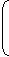100 x 76.50% = 16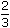%
 612 x 3 4

7.

The true discount on a bill due 9 months hence at 16% per annum is Rs. 189. The amount of the bill is:

 A. Rs. 1386 B. Rs. 1764 C. Rs. 1575 D. Rs. 2268

Explanation:

Let P.W. be Rs. x.

Then, S.I. on Rs. x at 16% for 9 months = Rs. 189.x x 16 x 9 x 1 = 189 or x = 1575. 12 100P.W. = Rs. 1575.Sum due = P.W. + T.D. = Rs. (1575 + 189) = Rs. 1764.

8.

A man buys a watch for Rs. 1950 in cash and sells it for Rs. 2200 at a credit of 1 year. If the rate of interest is 10% per annum, the man:

 A. gains Rs. 55 B. gains Rs. 50 C. loses Rs. 30 D. gains Rs. 30

Explanation:

S.P. = P.W. of Rs. 2200 due 1 year hence
 = Rs.2200 x 100100 + (10 x 1)
= Rs. 2000.Gain = Rs. (2000 - 1950) = Rs. 50.

9.

The true discount on Rs. 1760 due after a certain time at 12% per annum is Rs. 160. The time after which it is due is:

 A. 6 months B. 8 months C. 9 months D. 10 months

Explanation:

P.W. = Rs. (1760 -160) = Rs. 1600.S.I. on Rs. 1600 at 12% is Rs. 160.Time =100 x 160= 5 years =5 x 12months = 10 months. 1600 x 12 6 6

10.

The present worth of Rs. 2310 due 2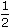years hence, the rate of interest being 15% per annum, is:

 A. Rs. 1750 B. Rs. 1680 C. Rs. 1840 D. Rs. 1443.75

P.W. = Rs.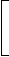100 x 2310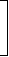= Rs. 1680.
 100 +15 x 52